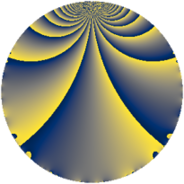# Properties

 Label 496.1.e.aLevel $496$ Weight $1$ Character orbit 496.e Self dual yes Analytic conductor $0.248$ Analytic rank $0$ Dimension $1$ Projective image $D_{3}$ CM discriminant -31 Inner twists $2$

# Related objects

Show commands: Magma / PariGP / SageMath

## Newspace parameters

comment: Compute space of new eigenforms

[N,k,chi] = [496,1,Mod(433,496)]

mf = mfinit([N,k,chi],0)

lf = mfeigenbasis(mf)

from sage.modular.dirichlet import DirichletCharacter

H = DirichletGroup(496, base_ring=CyclotomicField(2))

chi = DirichletCharacter(H, H._module([0, 0, 1]))

N = Newforms(chi, 1, names="a")

//Please install CHIMP (https://github.com/edgarcosta/CHIMP) if you want to run this code

chi := DirichletCharacter("496.433");

S:= CuspForms(chi, 1);

N := Newforms(S);

 Level: $$N$$ $$=$$ $$496 = 2^{4} \cdot 31$$ Weight: $$k$$ $$=$$ $$1$$ Character orbit: $$[\chi]$$ $$=$$ 496.e (of order $$2$$, degree $$1$$, not minimal)

## Newform invariants

comment: select newform

sage: f = N # Warning: the index may be different

gp: f = lf \\ Warning: the index may be different

 Self dual: yes Analytic conductor: $$0.247536246266$$ Analytic rank: $$0$$ Dimension: $$1$$ Coefficient field: $$\mathbb{Q}$$ Coefficient ring: $$\mathbb{Z}$$ Coefficient ring index: $$1$$ Twist minimal: no (minimal twist has level 31) Projective image: $$D_{3}$$ Projective field: Galois closure of 3.1.31.1 Artin image: $D_6$ Artin field: Galois closure of 6.0.61504.2

## $q$-expansion

comment: q-expansion

sage: f.q_expansion() # note that sage often uses an isomorphic number field

gp: mfcoefs(f, 20)

 $$f(q)$$ $$=$$ $$q - q^{5} + q^{7} + q^{9}+O(q^{10})$$ q - q^5 + q^7 + q^9 $$q - q^{5} + q^{7} + q^{9} + q^{19} - q^{31} - q^{35} - q^{41} - q^{45} - 2 q^{47} + q^{59} + q^{63} - 2 q^{67} + q^{71} + q^{81} - q^{95} - q^{97}+O(q^{100})$$ q - q^5 + q^7 + q^9 + q^19 - q^31 - q^35 - q^41 - q^45 - 2 * q^47 + q^59 + q^63 - 2 * q^67 + q^71 + q^81 - q^95 - q^97

## Character values

We give the values of $$\chi$$ on generators for $$\left(\mathbb{Z}/496\mathbb{Z}\right)^\times$$.

 $$n$$ $$63$$ $$65$$ $$373$$ $$\chi(n)$$ $$1$$ $$-1$$ $$1$$

## Embeddings

For each embedding $$\iota_m$$ of the coefficient field, the values $$\iota_m(a_n)$$ are shown below.

For more information on an embedded modular form you can click on its label.

comment: embeddings in the coefficient field

gp: mfembed(f)

Label $$\iota_m(\nu)$$ $$a_{2}$$ $$a_{3}$$ $$a_{4}$$ $$a_{5}$$ $$a_{6}$$ $$a_{7}$$ $$a_{8}$$ $$a_{9}$$ $$a_{10}$$
433.1
 0
0 0 0 −1.00000 0 1.00000 0 1.00000 0
 $$n$$: e.g. 2-40 or 990-1000 Significant digits: Format: Complex embeddings Normalized embeddings Satake parameters Satake angles

## Inner twists

Char Parity Ord Mult Type
1.a even 1 1 trivial
31.b odd 2 1 CM by $$\Q(\sqrt{-31})$$

## Twists

By twisting character orbit
Char Parity Ord Mult Type Twist Min Dim
1.a even 1 1 trivial 496.1.e.a 1
4.b odd 2 1 31.1.b.a 1
8.b even 2 1 1984.1.e.b 1
8.d odd 2 1 1984.1.e.a 1
12.b even 2 1 279.1.d.b 1
20.d odd 2 1 775.1.d.b 1
20.e even 4 2 775.1.c.a 2
28.d even 2 1 1519.1.c.a 1
28.f even 6 2 1519.1.n.a 2
28.g odd 6 2 1519.1.n.b 2
31.b odd 2 1 CM 496.1.e.a 1
36.f odd 6 2 2511.1.m.e 2
36.h even 6 2 2511.1.m.a 2
44.c even 2 1 3751.1.d.b 1
44.g even 10 4 3751.1.t.a 4
44.h odd 10 4 3751.1.t.c 4
124.d even 2 1 31.1.b.a 1
124.g even 6 2 961.1.e.a 2
124.i odd 6 2 961.1.e.a 2
124.j even 10 4 961.1.f.a 4
124.l odd 10 4 961.1.f.a 4
124.n odd 30 8 961.1.h.a 8
124.p even 30 8 961.1.h.a 8
248.b even 2 1 1984.1.e.a 1
248.g odd 2 1 1984.1.e.b 1
372.b odd 2 1 279.1.d.b 1
620.e even 2 1 775.1.d.b 1
620.m odd 4 2 775.1.c.a 2
868.c odd 2 1 1519.1.c.a 1
868.r even 6 2 1519.1.n.b 2
868.bj odd 6 2 1519.1.n.a 2
1116.v even 6 2 2511.1.m.e 2
1116.bk odd 6 2 2511.1.m.a 2
1364.h odd 2 1 3751.1.d.b 1
1364.bc odd 10 4 3751.1.t.a 4
1364.bi even 10 4 3751.1.t.c 4

By twisted newform orbit
Twist Min Dim Char Parity Ord Mult Type
31.1.b.a 1 4.b odd 2 1
31.1.b.a 1 124.d even 2 1
279.1.d.b 1 12.b even 2 1
279.1.d.b 1 372.b odd 2 1
496.1.e.a 1 1.a even 1 1 trivial
496.1.e.a 1 31.b odd 2 1 CM
775.1.c.a 2 20.e even 4 2
775.1.c.a 2 620.m odd 4 2
775.1.d.b 1 20.d odd 2 1
775.1.d.b 1 620.e even 2 1
961.1.e.a 2 124.g even 6 2
961.1.e.a 2 124.i odd 6 2
961.1.f.a 4 124.j even 10 4
961.1.f.a 4 124.l odd 10 4
961.1.h.a 8 124.n odd 30 8
961.1.h.a 8 124.p even 30 8
1519.1.c.a 1 28.d even 2 1
1519.1.c.a 1 868.c odd 2 1
1519.1.n.a 2 28.f even 6 2
1519.1.n.a 2 868.bj odd 6 2
1519.1.n.b 2 28.g odd 6 2
1519.1.n.b 2 868.r even 6 2
1984.1.e.a 1 8.d odd 2 1
1984.1.e.a 1 248.b even 2 1
1984.1.e.b 1 8.b even 2 1
1984.1.e.b 1 248.g odd 2 1
2511.1.m.a 2 36.h even 6 2
2511.1.m.a 2 1116.bk odd 6 2
2511.1.m.e 2 36.f odd 6 2
2511.1.m.e 2 1116.v even 6 2
3751.1.d.b 1 44.c even 2 1
3751.1.d.b 1 1364.h odd 2 1
3751.1.t.a 4 44.g even 10 4
3751.1.t.a 4 1364.bc odd 10 4
3751.1.t.c 4 44.h odd 10 4
3751.1.t.c 4 1364.bi even 10 4

## Hecke kernels

This newform subspace is the entire newspace $$S_{1}^{\mathrm{new}}(496, [\chi])$$.

## Hecke characteristic polynomials

$p$ $F_p(T)$
$2$ $$T$$
$3$ $$T$$
$5$ $$T + 1$$
$7$ $$T - 1$$
$11$ $$T$$
$13$ $$T$$
$17$ $$T$$
$19$ $$T - 1$$
$23$ $$T$$
$29$ $$T$$
$31$ $$T + 1$$
$37$ $$T$$
$41$ $$T + 1$$
$43$ $$T$$
$47$ $$T + 2$$
$53$ $$T$$
$59$ $$T - 1$$
$61$ $$T$$
$67$ $$T + 2$$
$71$ $$T - 1$$
$73$ $$T$$
$79$ $$T$$
$83$ $$T$$
$89$ $$T$$
$97$ $$T + 1$$